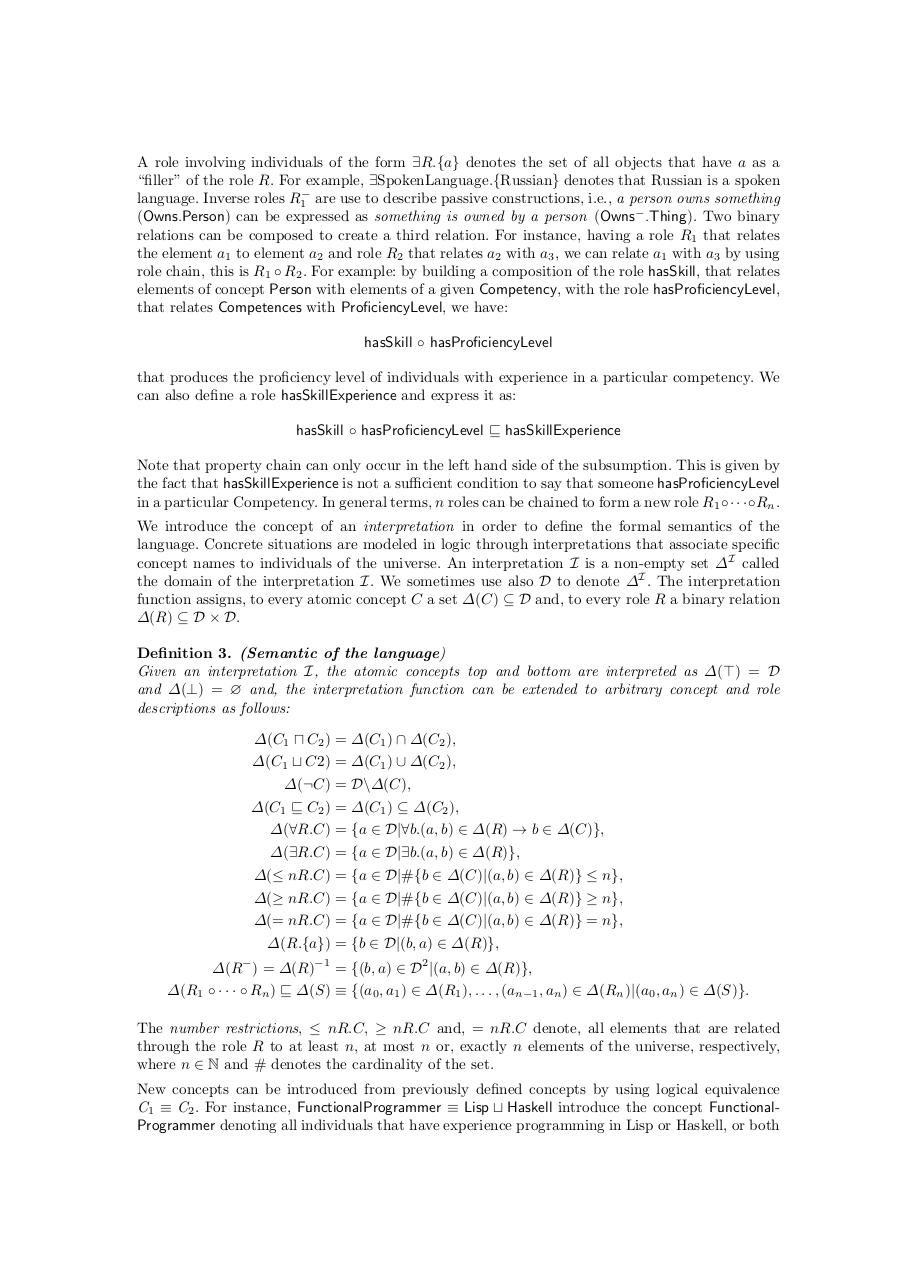# Matching Human Resources.pdfPage 1 2 3 4 5 6 7 8 9 10 11 12 13

#### Text preview

A role involving individuals of the form ∃R.{a} denotes the set of all objects that have a as a
“filler” of the role R. For example, ∃SpokenLanguage.{Russian} denotes that Russian is a spoken
language. Inverse roles R1− are use to describe passive constructions, i.e., a person owns something
(Owns.Person) can be expressed as something is owned by a person (Owns− .Thing). Two binary
relations can be composed to create a third relation. For instance, having a role R1 that relates
the element a1 to element a2 and role R2 that relates a2 with a3 , we can relate a1 with a3 by using
role chain, this is R1 ◦ R2 . For example: by building a composition of the role hasSkill, that relates
elements of concept Person with elements of a given Competency, with the role hasProficiencyLevel,
that relates Competences with ProficiencyLevel, we have:
hasSkill ◦ hasProficiencyLevel
that produces the proficiency level of individuals with experience in a particular competency. We
can also define a role hasSkillExperience and express it as:
hasSkill ◦ hasProficiencyLevel ⊑ hasSkillExperience
Note that property chain can only occur in the left hand side of the subsumption. This is given by
the fact that hasSkillExperience is not a sufficient condition to say that someone hasProficiencyLevel
in a particular Competency. In general terms, n roles can be chained to form a new role R1 ◦· · ·◦Rn .
We introduce the concept of an interpretation in order to define the formal semantics of the
language. Concrete situations are modeled in logic through interpretations that associate specific
concept names to individuals of the universe. An interpretation I is a non-empty set ∆I called
the domain of the interpretation I. We sometimes use also D to denote ∆I . The interpretation
function assigns, to every atomic concept C a set ∆(C) ⊆ D and, to every role R a binary relation
∆(R) ⊆ D × D.
Definition 3. (Semantic of the language)
Given an interpretation I, the atomic concepts top and bottom are interpreted as ∆(⊤) = D
and ∆(⊥) = ∅ and, the interpretation function can be extended to arbitrary concept and role
descriptions as follows:
∆(C1 ⊓ C2 ) = ∆(C1 ) ∩ ∆(C2 ),
∆(C1 ⊔ C2) = ∆(C1 ) ∪ ∆(C2 ),
∆(¬C) = D\∆(C),
∆(C1 ⊑ C2 ) = ∆(C1 ) ⊆ ∆(C2 ),
∆(∀R.C) = {a ∈ D|∀b.(a, b) ∈ ∆(R) → b ∈ ∆(C)},
∆(∃R.C) = {a ∈ D|∃b.(a, b) ∈ ∆(R)},
∆(≤ nR.C) = {a ∈ D|#{b ∈ ∆(C)|(a, b) ∈ ∆(R)} ≤ n},
∆(≥ nR.C) = {a ∈ D|#{b ∈ ∆(C)|(a, b) ∈ ∆(R)} ≥ n},
∆(= nR.C) = {a ∈ D|#{b ∈ ∆(C)|(a, b) ∈ ∆(R)} = n},
∆(R.{a}) = {b ∈ D|(b, a) ∈ ∆(R)},
∆(R ) = ∆(R)−1 = {(b, a) ∈ D2 |(a, b) ∈ ∆(R)},
∆(R1 ◦ · · · ◦ Rn ) ⊑ ∆(S) ≡ {(a0 , a1 ) ∈ ∆(R1 ), . . . , (an−1 , an ) ∈ ∆(Rn )|(a0 , an ) ∈ ∆(S)}.

The number restrictions, ≤ nR.C, ≥ nR.C and, = nR.C denote, all elements that are related
through the role R to at least n, at most n or, exactly n elements of the universe, respectively,
where n ∈ N and # denotes the cardinality of the set.
New concepts can be introduced from previously defined concepts by using logical equivalence
C1 ≡ C2 . For instance, FunctionalProgrammer ≡ Lisp ⊔ Haskell introduce the concept FunctionalProgrammer denoting all individuals that have experience programming in Lisp or Haskell, or both